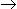# Aptitude - Numbers

### Exercise :: Numbers - General Questions

31.

On dividing a number by 5, we get 3 as remainder. What will the remainder when the square of the this number is divided by 5 ?

 A. 0 B. 1 C. 2 D. 4

Explanation:

Let the number be x and on dividing x by 5, we get k as quotient and 3 as remainder.x = 5k + 3x2 = (5k + 3)2

= (25k2 + 30k + 9)

= 5(5k2 + 6k + 1) + 4On dividing x2 by 5, we get 4 as remainder.

32.

How many 3-digit numbers are completely divisible 6 ?

 A. 149 B. 150 C. 151 D. 166

Explanation:

3-digit number divisible by 6 are: 102, 108, 114,... , 996

This is an A.P. in which a = 102, d = 6 and l = 996

Let the number of terms be n. Then tn = 996.a + (n - 1)d = 996102 + (n - 1) x 6 = 9966 x (n - 1) = 894(n - 1) = 149n = 150Number of terms = 150.

33.

How many natural numbers are there between 23 and 100 which are exactly divisible by 6 ?

 A. 8 B. 11 C. 12 D. 13 E. None of these

Explanation:

Required numbers are 24, 30, 36, 42, ..., 96

This is an A.P. in which a = 24, d = 6 and l = 96

Let the number of terms in it be n.

Then tn = 96a + (n - 1)d = 9624 + (n - 1) x 6 = 96(n - 1) x 6 = 72(n - 1) = 12n = 13

Required number of numbers = 13.

34.

How many of the following numbers are divisible by 3 but not by 9 ?
2133, 2343, 3474, 4131, 5286, 5340, 6336, 7347, 8115, 9276

 A. 5 B. 6 C. 7 D. None of these

Explanation:

Marking (/) those which are are divisible by 3 by not by 9 and the others by (X), by taking the sum of digits, we get:s

21339 (X)

234312 (/)

347418 (X)

41319 (X)

528621 (/)

534012 (/)

633618 (X)

734721 (/)

811515 (/)

927624 (/)

Required number of numbers = 6.

35.

 (963 + 476)2 + (963 - 476)2 = ? (963 x 963 + 476 x 476)

 A. 1449 B. 497 C. 2 D. 4 E. None of these

Explanation:

 Given Exp. = (a + b)2 + (a - b)2 = 2(a2 + b2) = 2 (a2 + b2) (a2 + b2)

36.

How many 3 digit numbers are divisible by 6 in all ?

 A. 149 B. 150 C. 151 D. 166

Explanation:

Required numbers are 102, 108, 114, ... , 996

This is an A.P. in which a = 102, d = 6 and l = 996

Let the number of terms be n. Then,

a + (n - 1)d = 996102 + (n - 1) x 6 = 9966 x (n - 1) = 894(n - 1) = 149n = 150.

37.

A 3-digit number 4a3 is added to another 3-digit number 984 to give a 4-digit number 13b7, which is divisible by 11. Then, (a + b) = ?

 A. 10 B. 11 C. 12 D. 15

Explanation:

``` 4 a 3  |
9 8 4  }  ==> a + 8 = b  ==>  b - a = 8
13 b 7  |
```

Also, 13 b7 is divisible by 11(7 + 3) - (b + 1) = (9 - b)(9 - b) = 0b = 9(b = 9 and a = 1)(a + b) = 10.

38.

8597 - ? = 7429 - 4358

 A. 5426 B. 5706 C. 5526 D. 5476 E. None of these

Explanation:

``` 7429          Let 8597 - x = 3071
-4358          Then,      x = 8597 - 3071
----                       = 5526
3071
----
```

39.

The smallest prime number is:

 A. 1 B. 2 C. 3 D. 4

Explanation:

The smallest prime number is 2.

40.

(12345679 x 72) = ?

 A. 88888888 B. 888888888 C. 898989898 D. 9999999998